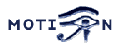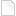Motion - Bug Report 2005x 05x 21x 105335

# BUG: Sometimes motion try to free an invalid memory area

Posted by KennethLavrsen from mailing list posting from ChristopheGRENIER

Sometimes motion try to free an invalid memory area

```for (j=0; config_params[j].param_name != NULL; j++) {
if (config_params[j].copy == copy_string) {
void **val;
val=(void *)cnt_list[i]+config_params[j].conf_value;
if (*val) {
/* FIXME BUG */
free(*val);
*val = NULL;
}
}
}
```

Except by removing the lines, I haven't found how to solve the problem.

## Test case

From follow up email.

```./motion
Processing thread 0 - config file motion.conf
Processing thread 1 - config file /home/motion/motion-3.2.1/thread1.conf
Processing thread 2 - config file /home/motion/motion-3.2.1/thread2.conf
Processing thread 3 - config file /home/motion/motion-3.2.1/thread3.conf
<Ctrl-C>
Motion terminating
*** glibc detected *** corrupted double-linked list: 0x092cf7b8 ***
Aborted

Same thing using valgrind
/usr/local/bin/valgrind --tool=memcheck --leak-check=full ./motion
==4886== Memcheck, a memory error detector for x86-linux.
==4886== Copyright (C) 2002-2005, and GNU GPL'd, by Julian Seward et al.
==4886== Using valgrind-2.4.0, a program supervision framework for x86-linux.
==4886== Copyright (C) 2000-2005, and GNU GPL'd, by Julian Seward et al.
==4886== For more details, rerun with: -v
==4886==
Processing thread 0 - config file motion.conf
Processing thread 1 - config file /home/motion/motion-3.2.1/thread1.conf
Processing thread 2 - config file /home/motion/motion-3.2.1/thread2.conf
Processing thread 3 - config file /home/motion/motion-3.2.1/thread3.conf
Motion terminating
==4886== Invalid free() / delete / delete[]
==4886==    at 0x1B90579D: free (vg_replace_malloc.c:152)
==4886==    by 0x804BD42: main (motion.c:1347)
==4886==  Address 0x1BBD2DA0 is not stack'd, malloc'd or (recently) free'd
==4886==
==4886== ERROR SUMMARY: 3 errors from 1 contexts (suppressed: 33 from 2)
==4886== malloc/free: in use at exit: 54894 bytes in 24 blocks.
==4886== malloc/free: 1872 allocs, 1851 frees, 87751488 bytes allocated.
==4886== For counts of detected errors, rerun with: -v
==4886== searching for pointers to 24 not-freed blocks.
==4886== checked 32346200 bytes.
==4886==
==4886==
==4886== 63 bytes in 3 blocks are definitely lost in loss record 1 of 7
==4886==    at 0x1B905CB2: realloc (vg_replace_malloc.c:196)
==4886==    by 0x804C2BC: myrealloc (motion.c:1406)
==4886==    by 0x805A275: netcam_start (netcam.c:1084)
==4886==    by 0x80510D9: vid_start (video.c:727)
==4886==    by 0x804C91D: motion_loop (motion.c:340)
==4886==    by 0x304FED: clone (in /lib/tls/libc-2.3.5.so)
==4886==
==4886==
==4886== 96 bytes in 3 blocks are definitely lost in loss record 2 of 7
==4886==    at 0x1B905298: malloc (vg_replace_malloc.c:130)
==4886==    by 0x805851B: netcam_url_match (netcam.c:116)
==4886==    by 0x8058640: netcam_url_parse (netcam.c:151)
==4886==    by 0x805A108: netcam_start (netcam.c:1045)
==4886==    by 0x80510D9: vid_start (video.c:727)
==4886==    by 0x804C91D: motion_loop (motion.c:340)
==4886==    by 0x304FED: clone (in /lib/tls/libc-2.3.5.so)
==4886==
==4886==
==4886== 111 bytes in 6 blocks are definitely lost in loss record 3 of 7
==4886==    at 0x1B905298: malloc (vg_replace_malloc.c:130)
==4886==    by 0x804B256: mymalloc (motion.c:1379)
==4886==    by 0x805AC31: strdupdelim (netcam_wget.c:249)
==4886==    by 0x805ACA6: http_process_type (netcam_wget.c:268)
==4886==    by 0x805A2BB: netcam_start (netcam.c:1095)
==4886==    by 0x80510D9: vid_start (video.c:727)
==4886==    by 0x804C91D: motion_loop (motion.c:340)
==4886==    by 0x304FED: clone (in /lib/tls/libc-2.3.5.so)
==4886==
==4886==
==4886== 204 bytes in 3 blocks are possibly lost in loss record 4 of 7
==4886==    at 0x1B905BC9: calloc (vg_replace_malloc.c:175)
==4886==    by 0x1B8F268A: _dl_allocate_tls (in /lib/ld-2.3.5.so)
==4886==    by 0x804BA91: main (motion.c:1288)
==4886==
==4886==
==4886== 38152 (252 direct, 37900 indirect) bytes in 3 blocks are definitely lost in loss record 5 of 7
==4886==    at 0x1B905298: malloc (vg_replace_malloc.c:130)
==4886==    by 0xC9B6A0: jpeg_get_small (in /usr/lib/libjpeg.so.62.0.0)
==4886==    by 0xC9B540: jinit_memory_mgr (in /usr/lib/libjpeg.so.62.0.0)
==4886==    by 0xC8C7EF: jpeg_CreateDecompress (in /usr/lib/libjpeg.so.62.0.0)
==4886==    by 0x805A441: netcam_start (netcam.c:1121)
==4886==    by 0x80510D9: vid_start (video.c:727)
==4886==    by 0x804C91D: motion_loop (motion.c:340)
==4886==    by 0x304FED: clone (in /lib/tls/libc-2.3.5.so)
==4886==
==4886==
==4886== 16268 bytes in 1 blocks are possibly lost in loss record 6 of 7
==4886==    at 0x1B905298: malloc (vg_replace_malloc.c:130)
==4886==    by 0xC9B6A0: jpeg_get_small (in /usr/lib/libjpeg.so.62.0.0)
==4886==    by 0xC9A7FB: (within /usr/lib/libjpeg.so.62.0.0)
==4886==    by 0xC8EA8D: (within /usr/lib/libjpeg.so.62.0.0)
==4886==    by 0xC8F9BF: (within /usr/lib/libjpeg.so.62.0.0)
==4886==    by 0xC8E1FF: (within /usr/lib/libjpeg.so.62.0.0)
==4886==    by 0xC8C919: jpeg_consume_input (in /usr/lib/libjpeg.so.62.0.0)
==4886==    by 0x805A473: netcam_start (netcam.c:1124)
==4886==    by 0x80510D9: vid_start (video.c:727)
==4886==    by 0x804C91D: motion_loop (motion.c:340)
==4886==
==4886== LEAK SUMMARY:
==4886==    definitely lost: 522 bytes in 15 blocks.
==4886==    indirectly lost: 37900 bytes in 5 blocks.
==4886==      possibly lost: 16472 bytes in 4 blocks.
==4886==    still reachable: 0 bytes in 0 blocks.
==4886==         suppressed: 0 bytes in 0 blocks.
==4886== Reachable blocks (those to which a pointer was found) are not shown.
==4886== To see them, rerun with: --show-reachable=yes

The line number may not match yours because I am working on a patch
to

Regards,

Christophe
```

Christophe also posted his config files. They are attached to this bug report.

## Environment

 Motion version: 3.2.1_snap17 ffmpeg version: N/A Shared libraries: ffmpeg, mysql, postgresql Server OS:

-- KennethLavrsen - 21 May 2005

## Fix record

The problem is the netcam_userpass that is freed in netcam.c and not pointed to NULL so in the loop to free all the string config values is freed twice. I've attached a patch to solve the problem .

-- AngelCarpintero - 23 May 2005

Added to the 3.2.1 sourcesn and the 3.1.20 sources. Thanks again Angel.

-- KennethLavrsen - 23 May 2005

### BugReportFormedit

 TopicTitle Sometimes motion try to free an invalid memory area BugStatus Released AssignedBugTo KennethLavrsen SubmittedBy ChristopheGRENIER
Topic attachments
I Attachment Action Size Date Who Commentdiff fix-freed-twice.diff manage 732 bytes 23 May 2005 - 13:29 AngelCarpinterogz motion_conf.tar.gz manage 5 K 21 May 2005 - 11:00 KennethLavrsen
Topic revision: r5 - 27 May 2005, KennethLavrsen
Copyright © 1999-2019 by the contributing authors. All material on this collaboration platform is the property of the contributing authors.
Please do not email Kenneth for support questions (read why). Use the Support Requests page or join the Mailing List.
This website only use harmless session cookies. See Cookie Policy for details. By using this website you accept the use of these cookies.